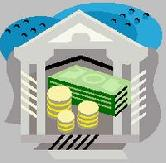Financial Terms Pure expectations theory

# Definition of Pure expectations theory## Pure expectations theory

A theory that asserts that the forward rates exclusively represent the expected
future rates. In other words, the entire term structure reflects the markets expectations of future short-term
rates. For example, an increasing sloping term structure implies increasing short-term interest rates. Related:
biased expectations theories

# Related Terms:

## Biased expectations theories

Related: pure expectations theory.

## Local expectations theory

A form of the pure expectations theory which suggests that the returns on bonds
of different maturities will be the same over a short-term investment horizon.

## Return-to-maturity expectations

A variant of pure expectations theory which suggests that the return that an
investor will realize by rolling over short-term bonds to some investment horizon will be the same as holding
a zero-coupon bond with a maturity that is the same as that investment horizon.

## Agency theory

The analysis of principal-agent relationships, wherein one person, an agent, acts on behalf of
anther person, a principal.

## Arbitrage Pricing Theory (APT)

An alternative model to the capital asset pricing model developed by
Stephen Ross and based purely on arbitrage arguments.

## Bubble theory

Security prices sometimes move wildly above their true values.

## Expectations hypothesis theories

Theories of the term structure of interest rates which include the pure
expectations theory, the liquidity theory of the term structure, and the preferred habitat theory. These theories
hold that each forward rate equals the expected future interest rate for the relevant period. These three theories
differ, however, on whether other factors also affect forward rates, and how.
expectations theory of forward exchange rates A theory of foreign exchange rates that holds that the
expected future spot foreign exchange rate t periods in the future equals the current t-period forward exchange
rate.## Homogenous expectations assumption

An assumption of Markowitz portfolio construction that investors
have the same expectations with respect to the inputs that are used to derive efficient portfolios: asset returns,
variances, and covariances.

## Liquidity theory of the term structure

A biased expectations theory that asserts that the implied forward
rates will not be a pure estimate of the market's expectations of future interest rates because they embody a

## Market segmentation theory or preferred habitat theory

A biased expectations theory that asserts that the
shape of the yield curve is determined by the supply of and demand for securities within each maturity sector.

## Modern portfolio theory

Principles underlying the analysis and evaluation of rational portfolio choices
based on risk-return trade-offs and efficient diversification.

## Normal backwardation theory

Holds that the futures price will be bid down to a level below the expected
spot price.

## Preferred habitat theory

A biased expectations theory that believes the term structure reflects the
expectation of the future path of interest rates as well as risk premium. However, the theory rejects the
assertion that the risk premium must rise uniformly with maturity. Instead, to the extent that the demand for
and supply of funds does not match for a given maturity range, some participants will shift to maturities
showing the opposite imbalances. As long as such investors are compensated by an appropriate risk premium
whose magnitude will reflect the extent of aversion to either price or reinvestment risk.

## Pure-discount bond

A bond that will make only one payment of principal and interest. Also called a zerocoupon
bond or a single-payment bond.

## Pure index fund

A portfolio that is managed so as to perfectly replicate the performance of the market portfolio.

## Pure yield pickup swap

Moving to higher yield bonds.## Rational expectations

The idea that people rationally anticipate the future and respond to what they see ahead.

## Static theory of capital structure

theory that the firm's capital structure is determined by a trade-off of the
value of tax shields against the costs of bankruptcy.

## theory of constraints (TOC)

a method of analyzing the bottlenecks
(constraints) that keep a system from achieving
higher performance; it states that production cannot take
place at a rate faster than the slowest machine or person
in the process

## expectations theory of exchange rates

theory that expected spot exchange rate equals the forward rate.

## pecking order theory

Firms prefer to issue debt rather than equity if internal finance is insufficient.

## random walk theory

Security prices change randomly, with no predictable trends or patterns.

Debt levels are chosen to balance interest tax shields against the costs of financial distress.

## Quantity Theory of Money

theory that velocity is constant, and so a change in money supply will change nominal income by the same percentage. Formalized by the equation Mv = PQ.

## Rational Expectations

The best forecasts that can be made given the data available and knowledge of how the economy operates. Rational expectations implies random errors, no systematic errors.

Belief that business cycles arise from real shocks to the economy, such as technology advances and natural resource discoveries, and have little to do with monetary policy.